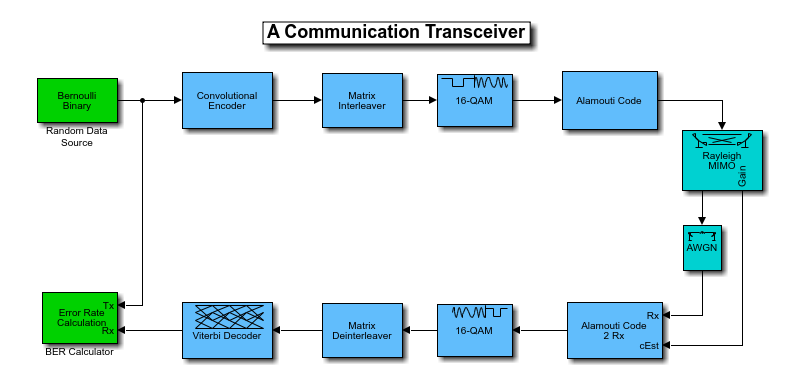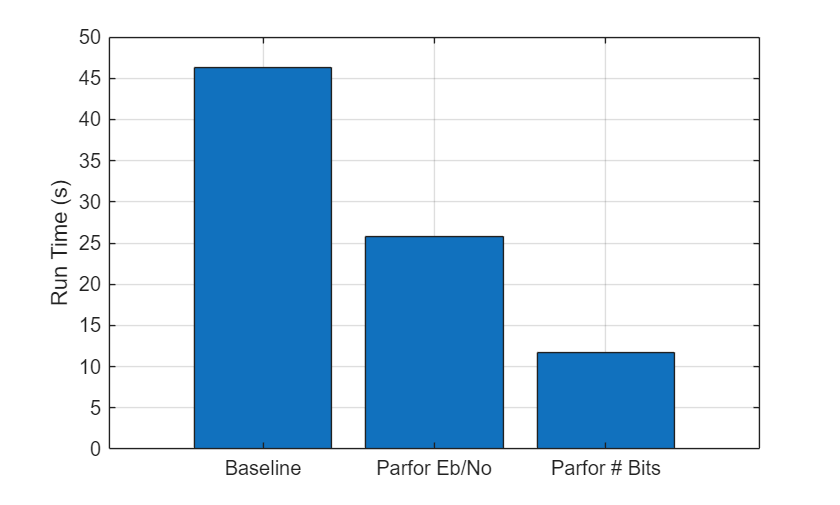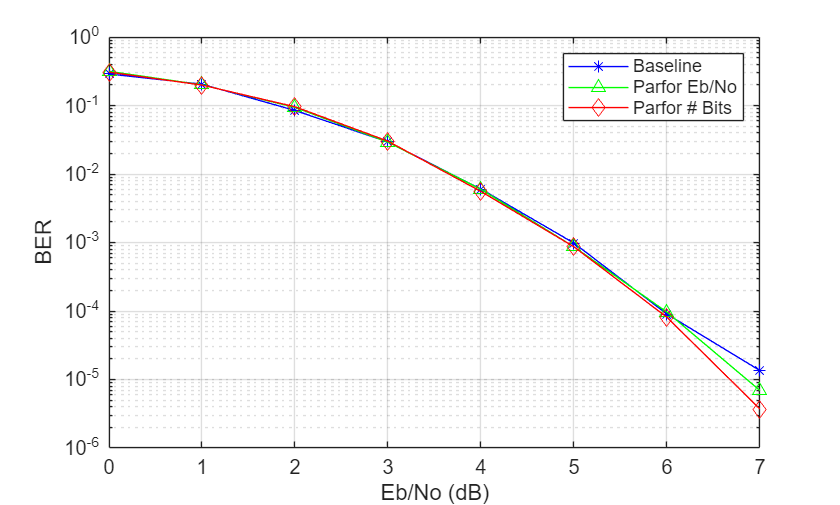# Simulation Acceleration Using MATLAB Coder and Parallel Computing Toolbox

This example shows two ways to accelerate the simulation of communications algorithms in MATLAB®. It showcases the runtime performance effects of using MATLAB to C code generation and parallel processing runs (using the MATLAB `parfor` (Parallel Computing Toolbox) function). For a comprehensive look at all possible acceleration techniques, see Accelerating MATLAB Algorithms and Applications article.

The combined effect of using these methods may speed up a typical simulation time by an order of magnitude. The difference is tantamount to running the simulation overnight or within just a few hours.

To run the MATLAB to C code generation section of this example, you must have MATLAB Coder™ product. To run the parallel processing section of this example, you must have Parallel Computing Toolbox™ product.

### Example Structure

This example examines various implementations of this transceiver system in MATLAB.This system is composed of a transmitter, a channel model, and a receiver. The transmitter processes the input bit stream with a convolutional encoder, an interleaver, a modulator, and a MIMO space-time block encoder (see [ 1 ], [ 2 ]). The transmitted signal is then processed by a 2x2 MIMO block fading channel and an additive white gaussian noise (AWGN) channel. The receiver processes its input signal with a 2x2 MIMO space-time block decoder, a demodulator, a deinterleaver, and a Viterbi decoder to recover the best estimate of the input bit stream at the receiver.

The example follows this workflow:

1. Create a function that runs the simulation algorithms

2. Use the MATLAB Profiler GUI to identify speed bottlenecks

3. Accelerate the simulation with MATLAB to C code generation

4. Achieve even faster simulation using parallel processing runs

### Create Function that Runs Simulation Algorithms

Start with a function that represents the first version or baseline implementation of this algorithm. The inputs to the `helperAccelBaseline` function are the ${E}_{b}/{N}_{o}$ value of the current frame (`EbNo`), minimum number of errors (`minNumErr`) and the maximum number of bits processed (`maxNumBits`). ${E}_{b}/{N}_{o}$ is the ratio of energy per bit to noise power spectral density. The function output is the bit error rate (BER) information for each ${E}_{b}/{N}_{o}$ point.

`type helperAccelBaseline`
```function ber = helperAccelBaseline(EbNo, minNumErr, maxNumBits) %helperAccelBaseline Simulate a communications link % BER = helperAccelBaseline(EBNO,MINERR,MAXBIT) returns the bit error % rate (BER) of a communications link that includes convolutional coding, % interleaving, QAM modulation, an Alamouti space-time block code, and a % MIMO block fading channel with AWGN. EBNO is the energy per bit to % noise power spectral density ratio (Eb/No) of the AWGN channel in dB, % MINERR is the minimum number of errors to collect, and MAXBIT is the % maximum number of simulated bits so that the simulations do not run % indefinitely if the Eb/No value is too high. % Copyright 2011-2021 The MathWorks, Inc. M = 16; % Modulation Order k = log2(M); % Bits per Symbol codeRate = 1/2; % Coding Rate adjSNR = convertSNR(EbNo,"ebno","BitsPerSymbol",k,"CodingRate",codeRate); trellis = poly2trellis(7,[171 133]); tblen = 32; dataFrameLen = 1998; % Add 6 zeros to terminate the convolutional code chanFrameLen=(dataFrameLen+6)/codeRate; permvec=[1:3:chanFrameLen 2:3:chanFrameLen 3:3:chanFrameLen]'; ostbcEnc = comm.OSTBCEncoder(NumTransmitAntennas=2); ostbcComb = comm.OSTBCCombiner(NumTransmitAntennas=2,NumReceiveAntennas=2); mimoChan = comm.MIMOChannel(MaximumDopplerShift=0,PathGainsOutputPort=true); berCalc = comm.ErrorRate; % Run Simulation ber = zeros(3,1); while (ber(3) <= maxNumBits) && (ber(2) < minNumErr) data = [randi([0 1],dataFrameLen,1);false(6,1)]; encOut = convenc(data,trellis); % Convolutional Encoder intOut = intrlv(double(encOut),permvec'); % Interleaver modOut = qammod(intOut,M,... 'InputType','bit'); % QAM Modulator stbcOut = ostbcEnc(modOut); % Alamouti Space-Time Block Encoder [chanOut, pathGains] = mimoChan(stbcOut); % 2x2 MIMO Channel chEst = squeeze(sum(pathGains,2)); rcvd = awgn(chanOut,adjSNR,'measured'); % AWGN channel stbcDec = ostbcComb(rcvd,chEst); % Alamouti Space-Time Block Decoder demodOut = qamdemod(stbcDec,M,... 'OutputType','bit'); % QAM Demodulator deintOut = deintrlv(demodOut,permvec'); % Deinterleaver decOut = vitdec(deintOut(:),trellis, ... % Viterbi Decoder tblen,'term','hard'); ber = berCalc(decOut(1:dataFrameLen),data(1:dataFrameLen)); end ```

As a starting point, measure the time it takes to run this baseline algorithm in MATLAB. Use the MATLAB timing functions (`tic` and `toc`) to record the elapsed runtime to complete processing of a for-loop that iterates over ${E}_{b}/{N}_{o}$ values from 0 to 7 dB.

```minEbNodB=0; maxEbNodB=7; EbNoVec = minEbNodB:maxEbNodB; minNumErr=100; maxNumBits=1e6; N=1; str='Baseline'; % Run the function once to load it into memory and remove overhead from % runtime measurements helperAccelBaseline(3,10,1e4); berBaseline=zeros(size(minEbNodB:maxEbNodB)); disp('Processing the baseline algorithm.');```
```Processing the baseline algorithm. ```
```tic; for EbNoIdx=1:length(EbNoVec) EbNo = EbNoVec(EbNoIdx); y=helperAccelBaseline(EbNo,minNumErr,maxNumBits); berBaseline(EbNoIdx)=y(1); end rtBaseline=toc;```

The result shows the simulation time (in seconds) of the baseline algorithm. Use this timing measurement to compare with subsequent accelerated simulation runtimes.

`helperAccelReportResults(N,rtBaseline,rtBaseline,str,str);`
```---------------------------------------------------------------------------------------------- Versions of the Transceiver | Elapsed Time (sec)| Acceleration Ratio 1. Baseline | 6.6191 | 1.0000 ---------------------------------------------------------------------------------------------- ```

### Identify Speed Bottlenecks by Using MATLAB Profiler App

Identify the processing bottlenecks and problem areas of the baseline algorithm by using the MATLAB Profiler. Obtain the profiler information by executing the following script:

```profile on y=helperAccelBaseline(6,100,1e6); profile off profile viewer```

The Profiler report presents the execution time for each function call of the algorithm. You can sort the functions according to their self-time in a descending order. The first few functions that the Profiler window depicts represent the speed bottleneck of the algorithm. In this case, the `vitdec` function is identified as the major speed bottleneck.

### Accelerate Simulation with MATLAB to C Code Generation

MATLAB Coder generates portable and readable C code from algorithms that are part of the MATLAB code generation subset. You can create a MATLAB executable (MEX) of the `helperAccelBaseline`, function because it uses functions and System objects that support code generation. Use the `codegen` (MATLAB Coder) function to compile the `helperAccelBaseline` function into a MEX function. After successful code generation by codegen, you will see a MEX file in the workspace that appends '_mex' to the function, `helperAccelBaseline_mex`.

`codegen('helperAccelBaseline.m','-args',{EbNo,minNumErr,maxNumBits})`
```Code generation successful. ```

Measure the simulation time for the MEX version of the algorithm. Record the elapsed time for running this function in the same for-loop as before.

```N=N+1; str='MATLAB to C code generation'; tag='Codegen'; helperAccelBaseline_mex(3,10,1e4); berCodegen=zeros(size(berBaseline)); disp('Processing the MEX function of the algorithm.');```
```Processing the MEX function of the algorithm. ```
```tic; for EbNoIdx=1:length(EbNoVec) EbNo = EbNoVec(EbNoIdx); y=helperAccelBaseline_mex(EbNo,minNumErr,maxNumBits); berCodegen(EbNoIdx)=y(1); end rt=toc;```

The results here show the MEX version of this algorithm runs faster than the baseline versions of the algorithm. The amount of acceleration achieved depends on the nature of the algorithm. The best way to determine the acceleration is to generate a MEX-function using MATLAB Coder and test the speedup firsthand. If your algorithm contains single-precision data types, fixed-point data types, loops with states, or code that cannot be vectorized, you are likely to see speedups. On the other hand, if your algorithm contains MATLAB implicitly multithreaded computations such as `fft` and `svd`, functions that call IPP or BLAS libraries, functions optimized for execution in MATLAB on a PC such as FFTs, or algorithms where you can vectorize the code, speedups are less likely.

`helperAccelReportResults(N,rtBaseline,rt,str,tag);`
```---------------------------------------------------------------------------------------------- Versions of the Transceiver | Elapsed Time (sec)| Acceleration Ratio 1. Baseline | 6.6191 | 1.0000 2. MATLAB to C code generation | 1.4821 | 4.4661 ---------------------------------------------------------------------------------------------- ```

### Achieve Even Faster Simulation Using Parallel Processing Runs

Utilize multiple cores to increase simulation acceleration by running tasks in parallel. Use parallel processing runs (`parfor` loops) in MATLAB to perform the work on the number of available workers. Parallel Computing Toolbox enables you to run different iterations of the simulation in parallel. Use the `gcp` (Parallel Computing Toolbox) function to get the current parallel pool. If a pool is available, the `gcp` opens the pool and reserves several MATLAB workers to execute iterations of a subsequent `parfor`-loop. In this example, six workers run locally on a MATLAB client machine.

`pool = gcp`
```pool = 0×0 Pool array with no properties. ```
```if isempty(pool) pool = parpool end```
```Starting parallel pool (parpool) using the 'local' profile ... Connected to the parallel pool (number of workers: 6). pool = ProcessPool with properties: Connected: true NumWorkers: 6 Busy: false Cluster: local AttachedFiles: {} AutoAddClientPath: true FileStore: [1x1 parallel.FileStore] ValueStore: [1x1 parallel.ValueStore] IdleTimeout: 30 minutes (30 minutes remaining) SpmdEnabled: true ```

#### Run Parallel Over Eb/No Values

Run ${E}_{b}/{N}_{o}$ points in parallel using six workers using a `parfor`-loop rather than a for-loop as used in the previous cases. Measure the simulation time.

```N=N+1; str='Parallel runs with parfor over Eb/No'; tag='Parfor Eb/No'; helperAccelBaseline_mex(3,10,1e4); berParfor1=zeros(size(berBaseline)); disp('Processing the MEX function of the algorithm within a parfor-loop.');```
```Processing the MEX function of the algorithm within a parfor-loop. ```
```tic; parfor EbNoIdx=1:length(EbNoVec) EbNo = EbNoVec(EbNoIdx); y=helperAccelBaseline_mex(EbNo,minNumErr,maxNumBits); berParfor1(EbNoIdx)=y(1); end rt=toc;```

The result adds the simulation time of the MEX version of the algorithm executing within a `parfor`-loop to the previous results. Note that by running the algorithm within a `parfor`-loop, the elapsed time to complete the simulation is shorter. The basic concept of a `parfor`-loop is the same as the standard MATLAB for-loop. The difference is that `parfor` divides the loop iterations into groups so that each worker executes some portion of the total number of iterations. Because several MATLAB workers can be computing concurrently on the same loop, a `parfor`-loop provides significantly better performance than a normal serial for-loop.

`helperAccelReportResults(N,rtBaseline,rt,str,tag);`
```---------------------------------------------------------------------------------------------- Versions of the Transceiver | Elapsed Time (sec)| Acceleration Ratio 1. Baseline | 6.6191 | 1.0000 2. MATLAB to C code generation | 1.4821 | 4.4661 3. Parallel runs with parfor over Eb/No | 1.2459 | 5.3127 ---------------------------------------------------------------------------------------------- ```

#### Run Parallel Over Number of Bits

In the previous section, the total simulation time is mainly determined by the highest ${E}_{b}/{N}_{o}$ point. You can further accelerate the simulations by dividing up the number of bits simulated for each ${E}_{b}/{N}_{o}$ point over the workers. Run each ${E}_{b}/{N}_{o}$ point in parallel using six workers using a `parfor`-loop. Measure the simulation time.

```N=N+1; str='Parallel runs with parfor over number of bits'; tag='Parfor # Bits'; helperAccelBaseline_mex(3,10,1e4); berParfor2=zeros(size(berBaseline)); disp('Processing the MEX function of the second version of the algorithm within a parfor-loop.');```
```Processing the MEX function of the second version of the algorithm within a parfor-loop. ```
```tic; % Calculate number of bits to be simulated on each worker minNumErrPerWorker = minNumErr / pool.NumWorkers; maxNumBitsPerWorker = maxNumBits / pool.NumWorkers; for EbNoIdx=1:length(EbNoVec) EbNo = EbNoVec(EbNoIdx); numErr = zeros(pool.NumWorkers,1); parfor w=1:pool.NumWorkers y=helperAccelBaseline_mex(EbNo,minNumErrPerWorker,maxNumBitsPerWorker); numErr(w)=y(2); numBits(w)=y(3); end berParfor2(EbNoIdx)=sum(numErr)/sum(numBits); end rt=toc;```

The result adds the simulation time of the MEX version of the algorithm executing within a `parfor`-loop where this time each worker simulates the same ${E}_{b}/{N}_{o}$ point. Note that by running this version within a `parfor`-loop we get the fastest simulation performance. The difference is that `parfor` divides the number of bits that needs to be simulated over the workers. This approach reduces the simulation time of even the highest ${E}_{b}/{N}_{o}$ value by evenly distributing load (specifically, the number of bits to simulate) over workers.

`helperAccelReportResults(N,rtBaseline,rt,str,tag);`
```---------------------------------------------------------------------------------------------- Versions of the Transceiver | Elapsed Time (sec)| Acceleration Ratio 1. Baseline | 6.6191 | 1.0000 2. MATLAB to C code generation | 1.4821 | 4.4661 3. Parallel runs with parfor over Eb/No | 1.2459 | 5.3127 4. Parallel runs with parfor over number of bits | 0.8802 | 7.5201 ---------------------------------------------------------------------------------------------- ```

### Summary

You can significantly speed up simulations of your communications algorithms with the combined effects of MATLAB to C code generation and Parallel processing runs.

• MATLAB to C code generation accelerates the simulation by locking-down datatypes and sizes of every variable and by reducing the overhead of the interpreted language that checks for the size and datatype of variables in every line of the code.

• Parallel processing runs can substantially accelerate simulation by computing different iterations of your algorithm concurrently across a number of MATLAB workers.

• Parallelizing each ${E}_{b}/{N}_{o}$ point individually can accelerate further by speeding up even the longest running ${E}_{b}/{N}_{o}$ point.

The following shows the run time of all four approaches as a bar graph. The results may vary based on the specific algorithm, available workers, and selection of minimum number of errors and maximum number of bits.

`results = helperAccelReportResults;`This plot shows the BER curves for the different simulation processing approaches match each other closely. For each plotted ${E}_{b}/{N}_{0}$ each of the four versions of the algorithm ran with the maximum number of input bits set to ten million (`maxNumBits`=1e7) and the minimum number of bit errors set to five thousand (`minNumErr`=5000).### Further Exploration

This example uses the `gcp` function to reserve several MATLAB workers that run locally on your MATLAB client machine. By modifying the parallel configurations, you can accelerate the simulation even further by running the algorithm on a larger cluster of workers that are not on your MATLAB client machine. For a description of how to manage and use parallel configurations, see the Discover Clusters and Use Cluster Profiles (Parallel Computing Toolbox) topic.

The following functions are used in this example.

### Selected References

1. S. M. Alamouti, "A simple transmit diversity technique for wireless communications," IEEE® Journal on Selected Areas in Communications, vol. 16, no. 8, pp. 1451-1458, Oct. 1998.

2. V. Tarokh, H. Jafarkhami, and A. R. Calderbank, "Space-time block codes from orthogonal designs," IEEE Transactions on Information Theory, vol. 45, no. 5, pp. 1456-1467, Jul. 1999.